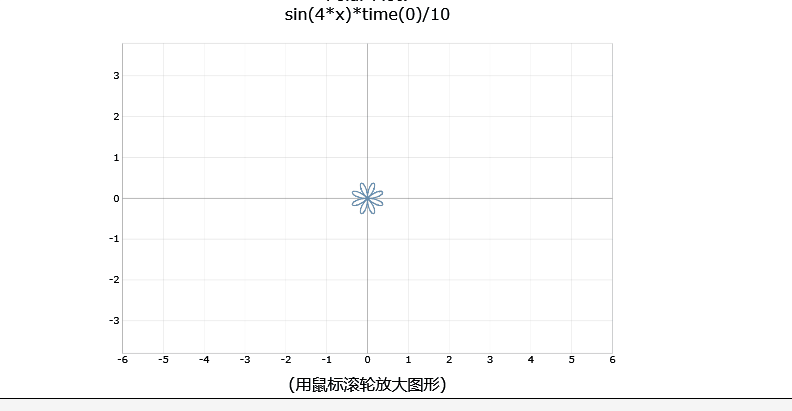﻿ math animation online software ﻿﻿ Fractional Calculus Computer Algebra System, Electrochemistry Software, Massage++=﻿

# math animation with online software MathHand.com 数学动画 1

### 1. Classication by plot function 按制图函数分类

Content
1. Classication by plot function 按制图函数分类:
1. plot 制图
2. polar plot 极坐标图
3. plot2D 制图2D
4. Web editor
5. plot3D 立体图
6. spin3D 旋转图3D
7. complex3D 复变函数图3D
8. parametric3D 参数立体图
9. implicit3D 隐函数图3D
2. Classication by appliaction 按应用分类:

## Classication of animation 动画分类

### Classication by plot function 按制图函数分类:

#### plot 制图

The plot( ) plots animation by adding the timer(x) into your formula.
1. sun rise 日升函数 semicircle(timer(3))
2. change the x value to minus, sunrise(-x) become sun down 日落函数 semicircle(timer(-3))
3. sin wave moving to right when x>0 向右正弦波 sin(x-timer(30))
4. sin wave moving to left when x<0 向左正弦波 sin(x-timer(-30))
5. sine runs after cosine as above two functions are combined 正弦波追余弦波
sin(x-timer(30))+1 and sin(x+timer(30))-1
6. blue peak grow slow and red peak grow fast 篮山峰长高慢 红山峰长高快
gauss(x+2)*timer(5) and gauss(x-2)*timer(10)
7. clock 时钟 timer(-x) and sqrt((9)-x*x)
8. tangent rides on sine wave 切线骑正弦波
sin(x+timer(30)) and cos(timer(30))*x+sin(timer(30))
9. define loving function, call it. 爱你函数
abs(x)^(2/3)+0.9*sqrt(3.3-x^2)*sin(pi*x*timer(30))
10. define a function of damped vibration wave, call it. 阻尼振动波
vibration(t_):=sin(10*x-timer(t))*exp(-0.1*x); vibration(30)

#### polar plot 极坐标图

The polarplot( ) plots animation by adding the timer(x) into your formula.
11. water wave 水波函数 polarplot( timer(6) )
12. Spiral of Archimedes 阿基米德螺线 polarplot( timer(x) )
13. heart become large 心大函数 polarplot( (1-sin(x))*timer(3) )
14. heart become small 心小函数 polarplot( (1-sin(x))*timer(-3) )
15. heart moving 心动函数 polarplot( 1-sin(x-timer(30)) )
16. flower spin 花旋转函数 polarplot( sin(2*x-timer(6)) )
17. flower spin 花旋转函数 polarplot( sin(3*x-timer(6)) )
18. flower spin 花旋转函数 polarplot( sin(4*x-timer(6)) )
19. flowering 开花函数 polarplot( sin(2*x)*timer(6) )
20. flowering 开花函数 polarplot( sin(3*x)*timer(6) )
21. flowering 开花函数 polarplot( sin(4*x)*timer(6) )
22. flowering 开花函数 polarplot( cos(2*x)*timer(6) )
23. flowering 开花函数 polarplot( cos(3*x)*timer(6) )
24. flowering 开花函数 polarplot( cos(4*x)*timer(6) )
25. heartbeat video 心动录像 heart.mp4
26. flowring video 开花录像 flowering.mp4#### plot2D 制图2D

The plot2D( ) plots animation on 4 ways:
1. click the auto-Axis checkbox.
4. On a device with a keyboard, try selecting one of the sliders and holding down a cursor key to see the patterns evolve continuously.

27. plot2D( )
28. plot2D?i=0.1*cos(x)*x&polar=on&animate=on 贝壳 shell 0.1*cos(x)*x
29. plot2D?i=x-t&t=10&polar=on&animate=on 顺时针旋转阿基米德螺线 Spiral of Archimedes x-t
30. plot2D?i=x+t&t=10&polar=on&animate=on 逆时针旋转阿基米德螺线 Spiral of Archimedes x+t
31. plot2D?i=cos(x-t)+%2b1&autoT=on 向右正弦波 cos(x-t)+1
32. plot2D?i=cos(x+%2bt)+%2b1&autoT=on 向左正弦波 cos(x+t)+1
33. Cornu Spiral the double Fresnel cosine and sine integrals fresnelC(x),fresnelS(x).
34. Cornu Spiral the Fresnel cosine and sine integrals fresnelC(x),fresnelS(x).
35. plot2D(fresnelC(x)) right-side spring 向右弹簧.
36. plot2D(-fresnelC(x)) left-side spring 向左弹簧.
37. plot2D(S(x),sin(x)-x) down-side spring 向下弹簧.
38. plot2D(S(x),sin(x)+x) up-side spring 向上弹簧.
39. plot2D(S(x),sin(x)-x) double spring 双弹簧.
40. sin(x-timer(9)) 向右正弦波
41. sin(x-timer(-9)) 向左正弦波
42. coloring butterfly 变色蝴蝶
43. coloring square 变色花环
44. Weierstrass Function
45. Unwrapping Circle
46. choreographies
47. x^2-y^2-t

#### Web editor

48. solar system 太阳系行星运行
49. taiji 太极图
50. taichi 八卦太极图
51. clock 时钟
52. html clock html 时钟
53. ccs clock ccs 时钟
54. heart beat 心跳
55. I love u 我爱你
56. percent 进度百分比
57. new year 新年
58. driving dog 会开车的狗
59. sea at night 夜里海水
60. fish jumping 鱼跳水
61. firework 烟花
62. solar in 2D 太阳系行星运行
63. cube

### Interactive 互动

64. water 可以升降的水
65. sea water 可以升降的海水
66. solar eclipse 日食
67. broke net 打破网
68. love tree 爱心树

#### plot3D 立体图

All 3D graphs are interactive, you can zoom, move, spin, etc.
69. solar 3D 互动太阳系
70. bianchi-pinkall-animation 指环
71. bianchi-pinkall-animation-zoomed 大指环

#### NOTICE Following 3D graph work only in Safari, Chrome, FireFox, etc.

The plot3D( ) plots 3D graph, which become animation by clicking the spin checkbox.
72. plot3D( )

#### spin3D 旋转图3D for 4 graphes in one plot

The spin3D plots spin of a function, implicit function, parametric function, a spacial curve, and its inverse in 3D graph.
1. a surface in space. 2. a curve in space. 3. cross-section. 4. positive value of a curve spins to make stereo.

73. spin3D
74. x*x
75. sin(x)
76. cos(x)
77. sin(x*x)
78. cos(x*x)
79. x^2+y^2=1
80. x^2-y^2-1

#### complex3D 复变函数图3D for 20 graphes in one plot

The complex3D( ) plots a function on the complex plane and space, which become animation by clicking the spin checkbox.

Here are some particularly nice examples by plot function complex3D( ) that show real and imaginary parts of complex function including special functions on complex axes and plane.

81. complex3D( )
More examples in complex function

#### parametric3D 函数图3D

82. parametric3D( )

#### implicit3D 隐函数图3D

83. implicit3D( )
More examples in Surface in 3D

#### in the space-time of dimension 4.

Have you ever tried to see objects in dimension 4? This is possible! Our space is in 3 dimensions. Adding the dimension of time, we get a space-time of dimension 4.

The objects in this space-time are therefore 3D objects which deform with the time. following this principle, this tool proposes to you the visualization of some of these objects as deforming 3D objects, with the aim of helping you to establish this vision in dimension 4.

The implicit3D( ) can plot a 4-dimensional (x,y,z,t) object in 3D space, which become animation by clicking the spin checkbox. You manually change the t value by slider.

84. x*x-y*y-z*z-t-1
More examples in Quadratic hypersurfaces in 4D

Next page =>

## Reference 参阅

• function graph 制图
• graphics 制图法
• example 例题

• Paul Masson
• Xiao Gang